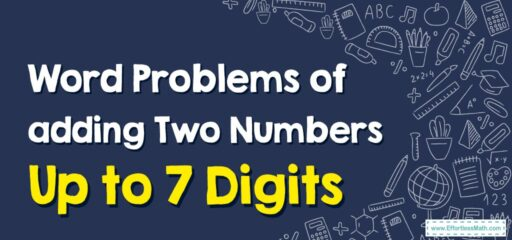# How to Solve Word Problems of Adding Two Numbers Up to 7 Digits

Solving word problems involving the addition of two numbers up to 7 digits can be straightforward once you understand the process. In a seven-digit number, the places are (from left to right): millions, hundred thousands, ten thousands, thousands, hundreds, tens, and ones.## A Step-by-step Guide to Solving Word Problems of Adding Two Numbers Up to 7 Digits

Here’s a step-by-step guide:

### Step 1: Read the problem carefully

Make sure you understand what the problem is asking. Look for the numbers you need to add and make sure you understand what these numbers represent in the context of the problem.

### Step 2: Identify the operation

In this case, the operation is addition, but always make sure you’re using the right operation for the problem at hand.

### Step 3: Write down the numbers

Write down the numbers you are going to add. Make sure you align them properly if you’re writing them one below the other, with each digit in the correct column (ones, tens, hundreds, thousands, etc.).

### Step 4: Perform the addition

Start adding from the rightmost digit (the ones place). If the sum is 10 or more, carry the ‘extra’ tens to the next column on the left.

### Step 5: Check your work

Let’s look at an example:

Problem: John has 3,456,789 apples in his orchard. Mary has 2,345,678 apples in her orchard. How many apples do they have in total?

Solution:

Write down the numbers, making sure to align the digits correctly:

  3456789 (John's apples)
+ 2345678 (Mary's apples)


  3456789
+ 2345678
----------
5802467


So, John and Mary have a total of 5,802,467 apples.

Remember to always re-read the problem and check your solution makes sense in the context of the problem.

### What people say about "How to Solve Word Problems of Adding Two Numbers Up to 7 Digits - Effortless Math: We Help Students Learn to LOVE Mathematics"?

No one replied yet.

X
30% OFF

Limited time only!

Save Over 30%

SAVE $5 It was$16.99 now it is \$11.99